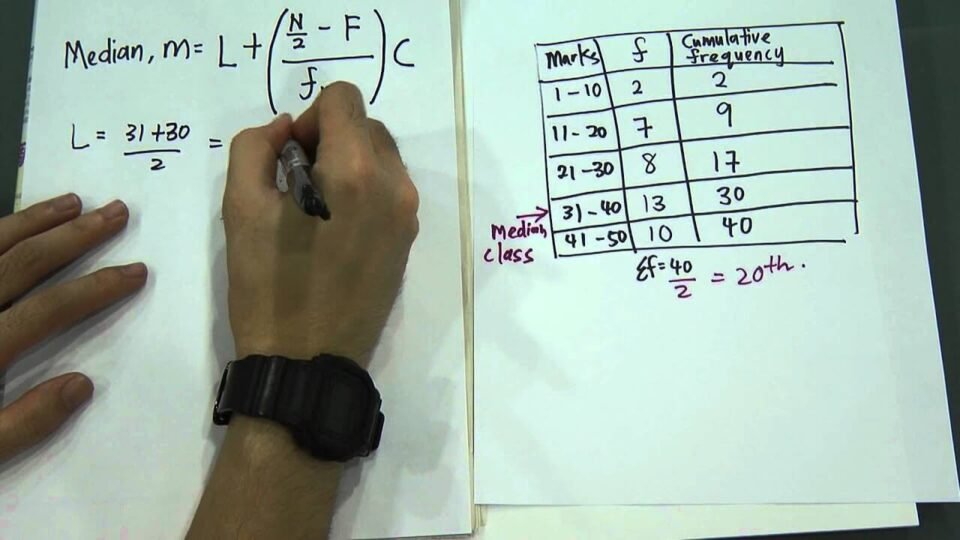General

# The Role Of Median In Statistics And The Process Of Calculation.

Introduction: In statistics, students need to deal with a large group of data. It helps them to find out how these data are associated with one another. For comparative study, it is crucial to plot these graphs and find out the relation. You may have heard the word “median” several times while dealing with statistics. It is nothing but the data that is present in the middle of the list. Many people confuse it with the term average or mean. However, the median formula just represents the data that lies exactly in the middle. With it, you can point out where the center of the graphical line is present.

## The concept of median

Contents

This is nothing but the data that is located exactly at the center of a set. You have to arrange them in a definite ascending or descending order for this purpose. When you find the median you will get two sets of data. One that belongs to the higher set and the one that belongs to the lower. The question becomes easy to solve when you set the data into groups. There you will find the frequency of each number as well. For ungrouped data, the students need to do a lot of calculations before beginning.

## Formulae for the median

There are different scenarios where you have to calculate the median. It depends on the set of data that students will deal with. The process may be longer if they are not arranged properly. Besides, you need to identify whether the total number of data is odd or even. It is your first job to calculate how many observations are present in the set. When you find it is odd, use the formula (n +1)/2 the position. It is quite easy to understand as you will be able to see it directly. For an even number the formula is [(n/2)term + {(n/2)+1}th]/2. N is nothing but how many observations you have in the question.

## Importance of median in our life

The term median is dependent on many other factors as well. You cannot calculate the average if you don’t know the median. This applies to some situations where you have too much ungrouped data. For example, there may be situations where the observations are even. You have a specific formula to solve such problems. But from where did the experts derive that formula while doing the sums? They just took the mean of the two central numbers and made a definite algorithm. The point where the median intersects is a part of the triangle centroid. Similarly, the term frequency allows you to simplify the entire sum. You don’t have to write the same data continuously as you can specify the frequency.

### How to approach these sums?

First, you need to see the case of ungrouped or grouped data. Just arrange them in a specific order to begin the sum properly. You can directly count and find the median for odd observations. To avoid any mistakes, add the number with one and divide by 2. In case the numbers are even, add the two middle parts first. When you make that half you will find the answer to your solution. For the ungrouped data, the total scenario will change. You have to calculate the cumulative frequency of the entire table first. The formula for this kind of sum is l+ (h/f)(n/2-c). To match the condition of a median set the cumulative frequency must be half the number of observations.

Students who don’t arrange the data often lose their marks in statistics. You cannot put the values directly into a formula without making the tables. Learn in detail about median by joining online math classes from online platforms such as Cuemath and know these rules properly.